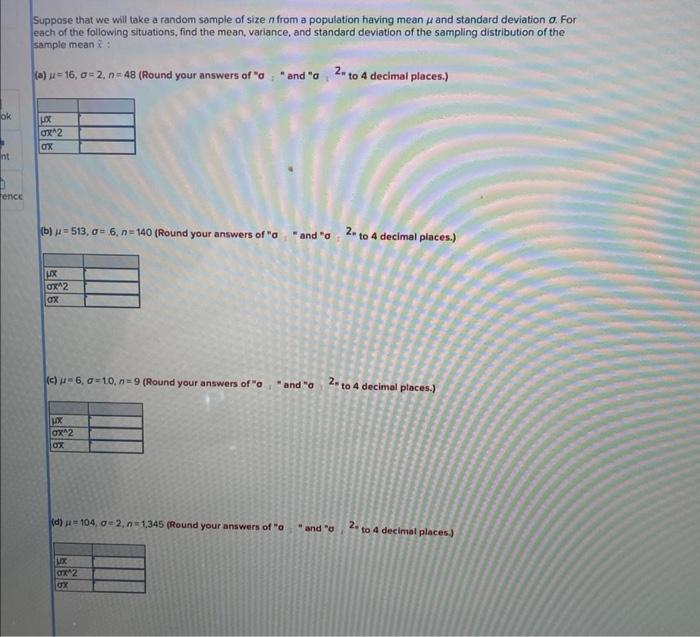Home / Expert Answers / Calculus / suppose-that-we-will-take-a-random-sample-of-size-n-from-a-population-having-mean-mu-a-pa127

# (Solved): Suppose that we will take a random sample of size $$n$$ from a population having mean $$\mu$$ a ...Suppose that we will take a random sample of size $$n$$ from a population having mean $$\mu$$ and standard deviation $$\sigma$$. For each of the following situations, find the mean, variance, and standard deviation of the sampling distribution of the sample mean $$\bar{x}$$ : (a) $$\mu=16, \sigma=2, n=48$$ (Round your answers of " $$a \quad$$ " and " $$a \quad 2 n$$ to 4 decimal places.) (b) $$\mu=513, \sigma=6, n=140$$ (Round your answers of " $$\sigma \quad$$ " and " $$\sigma \quad 2$$ n to 4 decimal places.) (c) $$\mu=6, \sigma=10, n=9$$ (Round your answers of " $$\sigma$$, " and " $$\sigma \quad 2=$$ to 4 decimal places.) (d) $$\mu=104, \sigma=2, n=1,345$$ (Round your answers of " $$a \quad$$ " and " $$a \quad 2 .$$ to 4 decimal places.)

We have an Answer from Expert